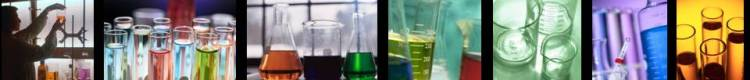Stoichiometry (Rules):

Rules for determining amount of substance reacting or formed in chemical equations:

1. Balance the equation.

2. Decide what quantity of each substance is given.  Write it below the substance (one line down if a mole quantity, two lines down if a laboratory quantity).

3. Decide what quantity you want to calculate.  Represent it with a question mark directly under the substance (one line down if a mole quantity, two lines down if a laboratory quantity).

4. Complete the diagram with arrows by going from the known laboratory quantity to moles, then to moles of unknown quantity, and finally to laboratory quantity of the unknown.  (Step(s) can be omitted if either or both the given quantity and the unknown quantity are moles.)

5. Calculate each quantity following the steps in the diagram.

How many liters (at STP) of NH3 are needed to react with 80.0 g of O2?

1)               4 NH3            +        5 O2  -->      4 NO       +      6 H2O

(step 2)

2)               moles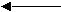moles

(step 3)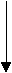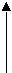(step 1)

? L                   80.0 g

3)  80.0 g O2 x 1 mole / 32.0 g  =  2.5 moles O2      (step 1)

From the balanced equation:  5 moles O2 consumed 4 moles of NH3 so, 2.5 moles O2 will consume 2 moles NH3      (step 2)

2 moles NH3 x 22.4 liters (STP) / 1 mole = 44.8 liters NH3 (STP)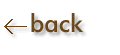Chemical Reactions of Copper and Percent Yield

Objective:  To gain familiarity with basic laboratory procedures, some chemistry of a typical transition element, and the concept of percent yield.

Apparatus and Chemicals

 0.5 g piece of no. 16 or no. 18 copper wire evaporating dish 250 mL beaker (2) weighing paper concentrated HNO3 6.0 M H2SO4 graduated cyliner granular zinc 3.0 M NaOH methanol carborundum boiling chips acetone stirring rod towel iron ring and ring stand balance wire gauze aluminum foil cut in 1-inch squares Bunsen burner concentrated HCl

Discussion:

Most chemical synthesis involve separation and purification of the desired product from unwanted side products.  Some methods of separation, such as filtration, sedimentation, decantation, extraction, and sublimation were discussed earlier.  This experiment is designed as a quantitative evaluation of your individual laboratory skills in carrying out some of these operations.  As the same time you will become more acquainted with two fundamental types of chemical reactions -- redox reactions and metathesis (double-displacement) reactions.  By means of these reactions, you will finally recover the copper sample with maximum efficiency.  The chemical reactions involved are the following.

Cu(s)  +  4 HNO3(aq)   ----->    Cu(NO3)2(aq)  +  2 NO2(g)  +  2 H2O(l)

Redox            

Cu(NO3)2(aq)  +  2 NaOH(aq)    ----->    Cu(OH)2(s)     +     2 NaNO3(aq)

Metathesis    

Cu(OH)2(s)   ----->    CuO(s)     +     H2O(g)

Dehydration     

CuO(s)     +     H2SO4(aq)    ----->    CuSO4(aq)     +     H2O(l)

Metathesis    

CuSO4(aq)     +     Zn(s)    ----->    ZnSO4(aq)     +     Cu(s)

Redox    

Each of these reactions proceeds to completion.  Metathesis reactions proceed to completion whenever one of the components is removed from the solution, such as in the formation of a gas or an insoluble precipitate (driving forces).  This is the case for reaction , , and , where in reactions  and  a gas and in reaction  an insoluble precipitate are formed.  Reaction  proceeds to completion because zinc has a lower ionization energy or oxidation potential that copper.

The objective in this experiment is to recover all of the copper you begin with in analytically pure form.  This is the test of your laboratory skills.

The percent yield of the copper can be expressed as the ratio of the recovered weight to initial weight, multiplied by 100: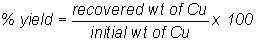Procedure:

• Weight approximately 0.500 g of no. 16 or no. 18 copper wire (1) to the nearest 0.0001 g and place it in a 250 mL beaker.  Add 4-5 mL of concentrated HNO3 to the beaker. IN THE HOOD.  After the reaction is complete, ass 100 mL distilled H2O.  Describe the reaction (6) as to color change, evolution of gas, and change in temperature (exothermic or endothermic) in the report sheet.

• Add 30 mL of 3.0 M NaOH to the solution in your beaker and describe the reaction (7).  Add two or three boiling chips and carefully heat the solution -- while stirring with a glass stirring rod -- just to the boiling point.  Describe the reaction on your report sheet (8).  Remove the boiling chips.

• Allow the black CuO to settle; then decant the supernantant liquid.  Add about 200 mL of very hot distilled water, stir, and then allow the CuO to settle.  Decant once more.  What are you removing by washing and decanting (9)?

• Add 15 mL of 6.0 M H2SO4.  What copper compound is present in the beaker now (10)?

Your instructor will tell you whether you should use zinc or aluminum for the reduction of Cu (II) in the following step.

A.  Zinc

In the hood, add 2.0 g of 30-mesh zinc metal all at once and stir until the supernatant liquid is colorless.  Describe the reaction on your report sheet (11).  What is present in solution (12)?  When gas evolution has become very slow, heat the solution gently (but do not boil) and allow it to cool.  What gas is formed in this reaction (13)?  How do you know (14)?

B.  Aluminum

In the hood, add several 1-inch squares of aluminum foil and a few drops of concentrated HCl.  Continue to add pieces of aluminum until the supernatant liquid is colorless.  Describe the reaction on your report sheet (11).  What is present in solution (12)?  What gas is formed in this reaction (13)?  How do you know (14)?

When gas evolution has ceased, decant the solution and transfer the precipitate to a preweighed porcelain evaporating dish (3).  Wash the precipitated copper with about 5 mL of distilled water, allow to settle, decant the solution, and repeat the process. What are you removing by washing (15)?  Wash the precipitate with about 5 mL of methanol (KEEP THE METHANOL AWAY FROM FLAMES _ IT IS FLAMMABLE!)  Allow the precipitate to settle, and decant the methanol.  (METHANOL IS ALSO EXTREMELY TOXIC:  AVOID BREATHING THE VAPORS AS MUCH AS POSSIBLE.)  Finally, wash the precipitate with about 5 mL of acetone (KEEP THE ACETONE AWAY FROM FLAMES - IT IS EXTREMELY FLAMMABLE!), allow the precipitate to settle, and decant the acetone from the precipitate.  Prepare a steam bath as illustrated and dry the product on your steam bath for at least 5 minutes.

Wipe the bottom of the evaporating dish with a towel, remove the boiling chips and weigh the evaporating dish plus copper (2).  Calculate the final a\weight of copper (4).  Compare the weight with your initial weight and calculate the percent yield (5).  What color is your copper sample (16)?  Is it uniform in appearance (17)?  Suggest possible sources of error in this experiment (18).

Pre-lab (Review Questions):

1.  Give an example, other than the ones listed in this experiment, of redox and metathesis reactions.

2.  When will reactions proceed to completion?

3.  Define percent yield in general terms.

4.  Name six methods of separating materials.

5.  Give criteria in terms of temperature changes for exothermic and endothermic reactions.

6.  If 1.65 g of Cu(NO3)2 are obtained from allowing 0.93 g of Cu to react with excess HNO3, what is the percent yield of the reaction?

7.  What is the maximum percent yield in any reaction?

8.  What is meant by the terms decantation and filtration?

9.  When Cu(OH)2(s) is heated, Copper (II) oxide and water are formed.  Write a balanced equation for the reaction.

10.  When sulfuric acid and copper (II) oxide are allowed to react, copper (II) sulfate and water are formed.  Write a balanced equation for this reaction.

11.  When copper (II) sulfate and aluminum are allowed to react, aluminum sulfate and copper are formed.  What kind of reaction is this?  Write a balanced equation for this reaction.

REPORT SHEET:  Chemical Reactions of Copper and Percent Yield

1.  Weight copper initial                                            _______________

2.  Weight of copper and evaporating dish            _______________

3.  Weight of evaporating dish                                _______________

4.  Weight of copper final                                        _______________

5.  % Yield (show calculations)                               _______________

6.  Describe the reaction  Cu(s)  +  HNO3(aq)  -->

7.  Describe the reaction Cu(NO3)2(aq)  +  NaOH(aq)  -->

8.  Describe the reaction Cu(OH)2(s)  -->

9.  What are you removing by this washing?

10.  What copper compound is present in the beaker?

11.  Describe the reaction CuSO4(aq)  +  Zn(s), or CuSO4(aq)  +  Al(s)

12.  What is present in solution?

13.  What is the gas?

14.  How do you know?

15.  What are you removing by washing?

16.  What color is your copper sample?

17.  Is it uniform in appearance?

18.  Suggest possible sources of error in this experiment.

POST LAB QUESTIONS

1.  If your percent yield of copper was greater than 100%, what are two plausible errors you may have made?

2.  Consider the combustion of methane, CH4:

CH4(g)  +  2 O2(g)       CO2(g)  +  2 H2O(g)

Suppose 2 mole of methane is allowed to react with 3 mol of oxygen.

a)  What is the limiting reagent?

b) How many moles of CO2 can be made from this mixture?  How many grams of CO2?

3.  Suppose 8.00 g of CH4 is allowed to burn in the presence of 6.00 g of oxygen.  How much (in grams) CH4, O2, CO2, and H2O remain after the reaction is complete?

4.  How many milliliters of 6.0 M H2SO4 are required to react with 0.80 g of CuO according to Equation ?

5.  If 2.00 g of Zn is allowed to react with 1.75 g of CuSO4 according to Equation , how many grams of Zn will remain after the reaction is complete?

6.  What is meant by the term limiting reagent?